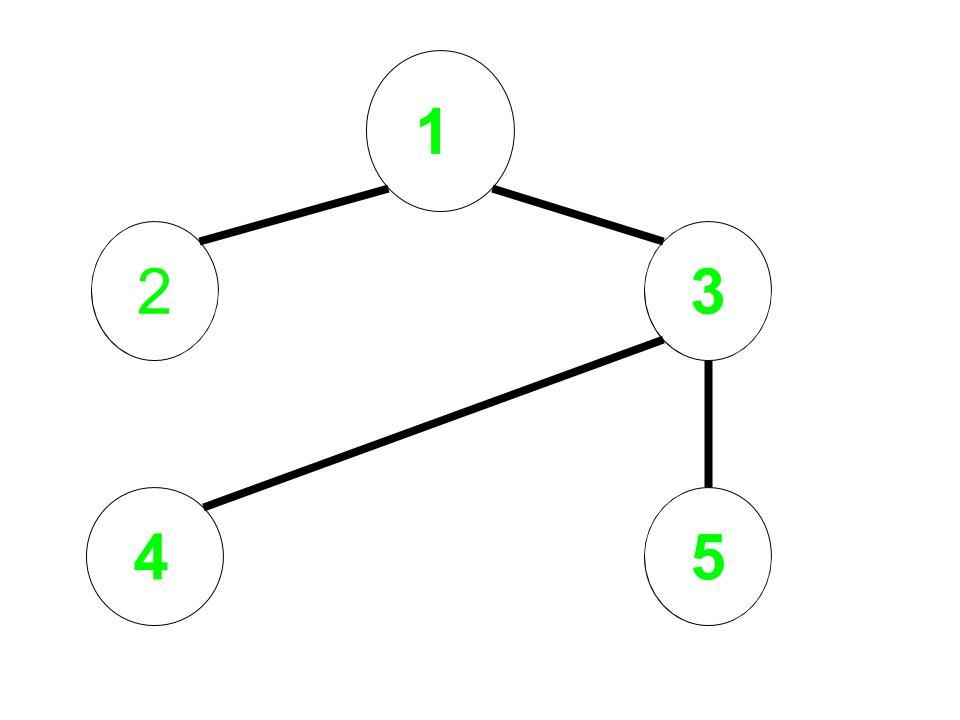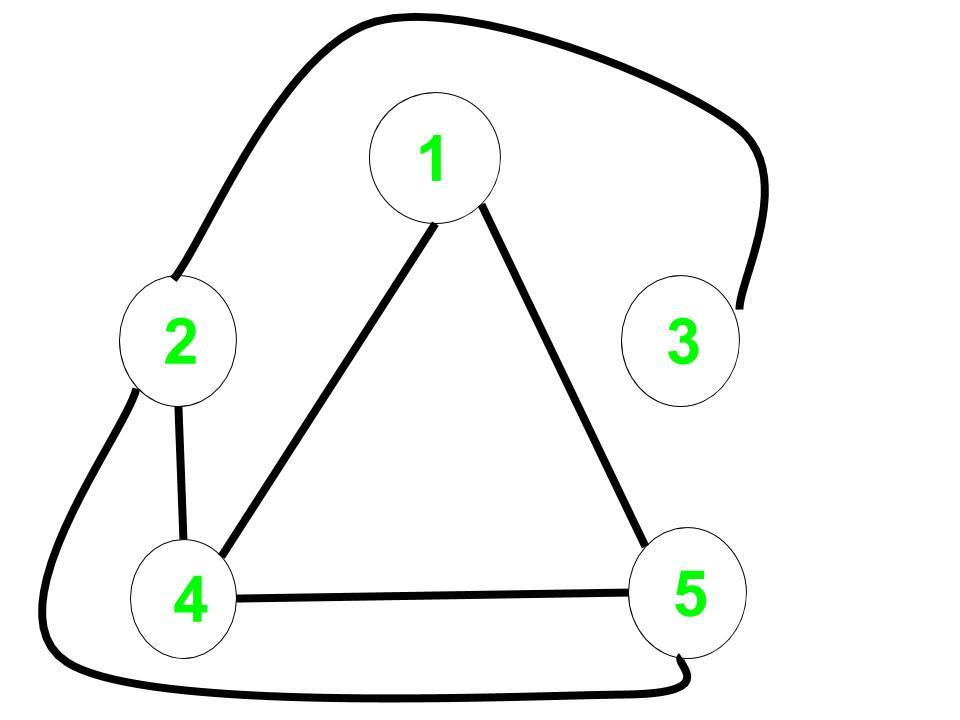# Shortest path in a complement graph

• Difficulty Level : Hard
• Last Updated : 28 Jan, 2021

Given an undirected non-weighted graph G. For a given node start return the shortest path that is the number of edges from start to all the nodes in the complement graph of G.

Complement Graph is a graph such that it contains only those edges which are not present in the original graph.

Examples:

Input: Undirected Edges = (1, 2), (1, 3), (3, 4), (3, 5), Start = 1
Output: 0 2 3 1 1
Explanation:
Original Graph:Complement Graph:The distance from 1 to every node in the complement graph are:
1 to 1 = 0,
1 to 2 = 2,
1 to 3 = 3,
1 to 4 = 1,
1 to 5 = 1

Naive Approach: A Simple solution will be to create the complement graph and use Breadth-First Search on this graph to find the distance to all the nodes.
Time complexity: O(n2) for creating the complement graph and O(n + m) for breadth first search.
Efficient Approach: The idea is to use Modified Breadth-First Search to calculate the answer and then there is no need to construct the complement graph.

• For each vertex or node, reduce the distance of a vertex which is a complement to the current vertex and has not been discovered yet.
• For the problem, we have to observe that if the Graph is Sparse then the undiscovered nodes will be visited very fast.

Below is the implementation of the above approach:

## C++

 `// C++ implementation to find the` `// shortest path in a complement graph`   `#include ` `using` `namespace` `std;`   `const` `int` `inf = 100000;`   `void` `bfs(``int` `start, ``int` `n, ``int` `m,` `         ``map, ``int``> edges)` `{` `    ``int` `i;`   `    ``// List of undiscovered vertices` `    ``// initially it will contain all` `    ``// the vertices of the graph` `    ``set<``int``> undiscovered;`   `    ``// Distance will store the distance` `    ``// of all vertices from start in the` `    ``// complement graph` `    ``vector<``int``> distance_node(10000);`   `    ``for` `(i = 1; i <= n; i++) {`   `        ``// All vertices are undiscovered` `        ``undiscovered.insert(i);`   `        ``// Let initial distance be infinity` `        ``distance_node[i] = inf;` `    ``}`   `    ``undiscovered.erase(start);` `    ``distance_node[start] = 0;` `    ``queue<``int``> q;`   `    ``q.push(start);`   `    ``// Check if queue is not empty and the` `    ``// size of undiscovered vertices` `    ``// is greater than 0` `    ``while` `(undiscovered.size() && !q.empty()) {` `        ``int` `cur = q.front();` `        ``q.pop();`   `        ``// Vector to store all the complement` `        ``// vertex to the current vertex` `        ``// which has not been` `        ``// discovered or visited yet.` `        ``vector<``int``> complement_vertex;`   `        ``for` `(``int` `x : undiscovered) {` `          `  `            ``if` `(edges.count({ cur, x }) == 0 && ` `                ``edges.count({ x, cur })==0)` `                ``complement_vertex.push_back(x);` `        ``}` `        ``for` `(``int` `x : complement_vertex) {`   `            ``// Check if optimal change` `            ``// the distance of this` `            ``// complement vertex` `            ``if` `(distance_node[x]` `                ``> distance_node[cur] + 1) {` `                ``distance_node[x]` `                    ``= distance_node[cur] + 1;` `                ``q.push(x);` `            ``}`   `            ``// Finally this vertex has been` `            ``// discovered so erase it from` `            ``// undiscovered vertices list` `            ``undiscovered.erase(x);` `        ``}` `    ``}` `    ``// Print the result` `    ``for` `(``int` `i = 1; i <= n; i++)` `        ``cout << distance_node[i] << ``" "``;` `}`   `// Driver code` `int` `main()` `{` `    ``// n is the number of vertex` `    ``// m is the number of edges` `    ``// start - starting vertex is 1` `    ``int` `n = 5, m = 4;`   `    ``// Using edge hashing makes the` `    ``// algorithm faster and we can` `    ``// avoid the use of adjacency` `    ``// list representation` `    ``map, ``int``> edges;`   `    ``// Initial edges for` `    ``// the original graph` `    ``edges[{ 1, 3 }] = 1,` `                 ``edges[{ 3, 1 }] = 1;` `    ``edges[{ 1, 2 }] = 1,` `                 ``edges[{ 2, 1 }] = 1;` `    ``edges[{ 3, 4 }] = 1,` `                 ``edges[{ 4, 3 }] = 1;` `    ``edges[{ 3, 5 }] = 1,` `                 ``edges[{ 5, 3 }] = 1;`   `    ``bfs(1, n, m, edges);`   `    ``return` `0;` `}`

## Java

 `// Java implementation to find the ` `// shortest path in a complement graph ` `import` `java.io.*;` `import` `java.util.*;`   `class` `GFG{` `    `  `// Pair class is made so as to` `// store the edges between nodes` `static` `class` `Pair` `{` `    ``int` `left;` `    ``int` `right;` `    `  `    ``public` `Pair(``int` `left, ``int` `right)` `    ``{` `        ``this``.left = left;` `        ``this``.right = right;` `    ``}` `    `  `    ``// We need to override hashCode so that ` `    ``// we can use Set's properties like contains()` `    ``@Override` `    ``public` `int` `hashCode()` `    ``{` `        ``final` `int` `prime = ``31``;` `        ``int` `result = ``1``;` `        ``result = prime * result + left;` `        ``result = prime * result + right;` `        ``return` `result;` `    ``}`   `    ``@Override` `    ``public` `boolean` `equals( Object other ) ` `    ``{` `        ``if` `(``this` `== other){``return` `true``;}` `        ``if` `(other ``instanceof` `Pair) ` `        ``{` `            ``Pair m = (Pair)other;` `            ``return` `this``.left == m.left &&` `                  ``this``.right == m.right;` `        ``}` `        ``return` `false``;` `    ``}` `}`   `public` `static` `void` `bfs(``int` `start, ``int` `n, ``int` `m, ` `                       ``Set edges) ` `{ ` `    ``int` `i; `   `    ``// List of undiscovered vertices ` `    ``// initially it will contain all ` `    ``// the vertices of the graph ` `    ``Set undiscovered = ``new` `HashSet<>(); `   `    ``// Distance will store the distance ` `    ``// of all vertices from start in the ` `    ``// complement graph ` `    ``int``[] distance_node = ``new` `int``[``1000``]; `   `    ``for``(i = ``1``; i <= n; i++)` `    ``{ `   `        ``// All vertices are undiscovered initially` `        ``undiscovered.add(i); `   `        ``// Let initial distance be maximum value ` `        ``distance_node[i] = Integer.MAX_VALUE; ` `    ``} ` `    `  `    ``// Start is discovered ` `    ``undiscovered.remove(start);` `    `  `    ``// Distance of the node to itself is 0` `    ``distance_node[start] = ``0``; ` `    `  `    ``// Queue used for BFS` `    ``Queue q = ``new` `LinkedList<>();`   `    ``q.add(start); `   `    ``// Check if queue is not empty and the ` `    ``// size of undiscovered vertices ` `    ``// is greater than 0 ` `    ``while` `(undiscovered.size() > ``0` `&& !q.isEmpty()) ` `    ``{ ` `        `  `        ``// Current node` `        ``int` `cur = q.peek(); ` `        ``q.remove(); `   `        ``// Vector to store all the complement ` `        ``// vertex to the current vertex ` `        ``// which has not been ` `        ``// discovered or visited yet. ` `        ``Listcomplement_vertex = ``new` `ArrayList<>(); `   `        ``for``(``int` `x : undiscovered)` `        ``{ ` `            ``Pair temp1 = ``new` `Pair(cur, x);` `            ``Pair temp2 = ``new` `Pair(x, cur);` `            `  `            ``// Add the edge if not already present` `            ``if` `(!edges.contains(temp1) && ` `                ``!edges.contains(temp2))` `            ``{ ` `                ``complement_vertex.add(x); ` `            ``}` `        ``} ` `        `  `        ``for``(``int` `x : complement_vertex)` `        ``{ ` `            `  `            ``// Check if optimal change ` `            ``// the distance of this ` `            ``// complement vertex ` `            ``if` `(distance_node[x] > ` `                ``distance_node[cur] + ``1``) ` `            ``{ ` `                ``distance_node[x] = ` `                ``distance_node[cur] + ``1``; ` `                ``q.add(x); ` `            ``} `   `            ``// Finally this vertex has been ` `            ``// discovered so erase it from ` `            ``// undiscovered vertices list ` `            ``undiscovered.remove(x); ` `        ``} ` `    ``} ` `    `  `    ``// Print the result ` `    ``for``(i = ``1``; i <= n; i++) ` `        ``System.out.print(distance_node[i] + ``" "``); ` `} ` `    `  `// Driver code ` `public` `static` `void` `main(String[] args) ` `{ ` `    `  `    ``// n is the number of vertex ` `    ``// m is the number of edges ` `    ``// start - starting vertex is 1 ` `    ``int` `n = ``5``, m = ``4``; `   `    ``// Using edge hashing makes the ` `    ``// algorithm faster and we can ` `    ``// avoid the use of adjacency ` `    ``// list representation ` `    ``Set edges = ``new` `HashSet<>(); `   `    ``// Initial edges for ` `    ``// the original graph ` `    ``edges.add(``new` `Pair(``1``, ``3``)); ` `    ``edges.add(``new` `Pair(``3``, ``1``));` `    ``edges.add(``new` `Pair(``1``, ``2``));` `    ``edges.add(``new` `Pair(``2``, ``1``));` `    ``edges.add(``new` `Pair(``3``, ``4``));` `    ``edges.add(``new` `Pair(``4``, ``3``));` `    ``edges.add(``new` `Pair(``3``, ``5``)) ;` `    ``edges.add(``new` `Pair(``5``, ``3``));` `    ``Pair t = ``new` `Pair(``1``, ``3``);`   `    ``bfs(``1``, n, m, edges); ` `}` `} `   `// This code is contributed by kunalsg18elec`

Output:

`0 2 3 1 1`

Time complexity: O(V+E)
Auxiliary Space: O(V)

My Personal Notes arrow_drop_up
Recommended Articles
Page :